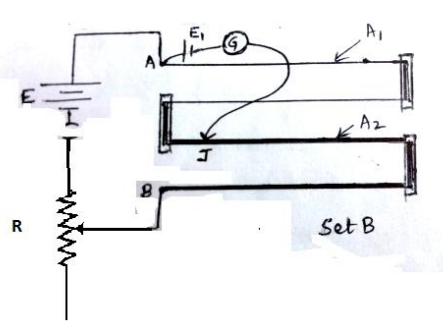# NEET Physics Current Electricity Questions SolvedBOARD

You are given two sets of potentiometer circuit to measure the emf E1 of a cell.

Set A: consists of a potentiometer wire of a material of resistivity ρ1, area of cross-section A1 and length l.

Set B: consists of a potentiometer of two composite wires of equal lengths l/2 each, of resistivity ρ1, ρ2 and area of cross-section A1, A2 respectively.

(i) Find the relation between resistivity of the two wires with respect to their area of cross section, if the current flowing in the two sets is same.

(ii) Compare the balancing length obtained in the two sets.                           (3) mark12 (a)  I =$\frac{\epsilon }{R+\frac{{\rho }_{1}l}{A1}}$

for Set A

I =$\frac{\epsilon }{R+\frac{{\rho }_{1}}{A1}}$

for set B

Equating the above two expressions and simplifying = $\frac{{\rho }_{1}}{A1}$                       (1/2) mark

(b) Potential gradient of the potentiometer wire for set A, K= I

Potential drop across the potentiometer wire in set B

 V = $I×\left(\frac{{\rho }_{1}l}{A1}=\frac{{\rho }_{2}l}{2A2}\right)$ V = $\frac{1}{2}\left(\frac{{\rho }_{1}}{A1}+\frac{{\rho }_{2}}{A2}\right)l$ (1/2) K’ =$\frac{1}{2}\left(\frac{{\rho }_{1}}{A1}+\frac{{\rho }_{2}}{A2}\right)$ , using the condition obtained in part (i) (1/2)

K’= I$\frac{{\rho }_{1}}{A1}$ , which is equal to K.

Therefore, balancing length obtained in the two sets is same.             (1/2)   mark

Difficulty Level:

Crack NEET with Online Course - Free Trial (Offer Valid Till September 24, 2019)